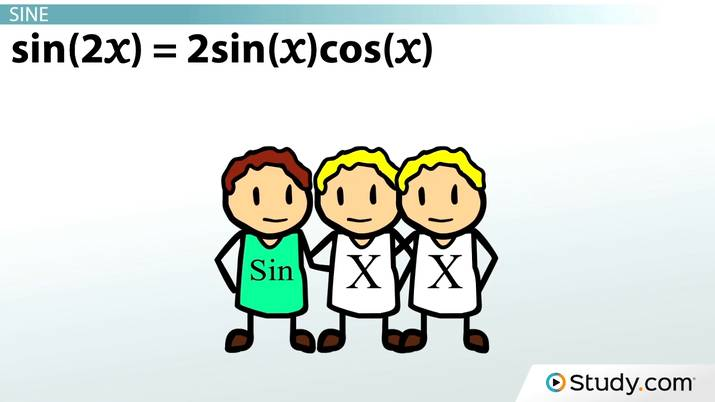The Double Angle Formula

Lesson Transcript
Instructor: Jeff Calareso

Jeff teaches high school English, math and other subjects. He has a master's degree in writing and literature.

In trigonometry, a double angle is twice as large as a common angle. Learn how to calculate the double angle formula, explore trigonometry twins for sine, cosine, and tangent, and apply this information in practice problems using the double angle formula. Updated: 09/28/2021

Trigonometry Twins

Let's say you're angle x. You're a happy angle. Life is good. You've got your home in a right triangle. And you have your straightforward sine, cosine and tangent friends, and when you get together with them, you know your values.

With sine, you're the opposite over the hypotenuse. With cosine, you're the adjacent over the hypotenuse. And with tangent, you're the opposite over the adjacent. SOH CAH TOA, nice and simple.

Then, one day, you find out you have a long-lost twin. Suddenly, you're not just x, you're 2x. Your world no longer makes sense. Most relevant to this lesson, your sine, cosine and tangent friends are confused. Fortunately, there are formulas for each of your friends to help. The double angle formulas are formulas that define the relationship between a trigonometric value and the double of the original angle. So take your twin and your old trigonometry friends and let's figure this out.An error occurred trying to load this video.

Try refreshing the page, or contact customer support.

Coming up next: Converting Between Radians and Degrees

You're on a roll. Keep up the good work!

Replay
Your next lesson will play in 10 seconds
• 0:05 Trigonometry Twins
• 1:09 Sine
• 2:57 Cosine
• 4:18 Tangent
• 6:05 Practice Problems
• 9:11 Lesson Summary
Save Save

Want to watch this again later?

Timeline
Autoplay
Autoplay
Speed Speed

Sine

Let's start with the double angle formula for sine. It's sin(2x) = 2sin(x) cos(x). Wait, how can that be? Why couldn't we just find the sine of x and then double that? Let's try it. Here's a triangle where x is 30. What's sin30? 1/2. Okay, so what's 2 * 1/2? 1. Is that the same as the sine of 60? No. The sine of 60 is (root 3) / 2. This is why your friend sine is so upset.

Let's test our double angle formula with the same angle. So sin(2x) is sin60. For our double angle formula to be true, sin60 = 2sin(30) cos(30). Sin60, again, is (root 3) / 2. Sin30 is still ½, and the cosine of 30: (root 3) / 2. So we have 2 * 1/2 * (root 3) / 2. These twos cancel and we're left with (root 3) / 2 = (root 3) / 2. That'll put sine at ease.

So, where does sin(2x) = 2sin(x) cos(x) come from? It comes from the summation formula for sine. If we didn't have 2x but had x and y, then sin(x + y) = sin(x) cos(y) + sin(y) cos(x). If those ys become xs, you have sin(x) cos(x) + sin(x) cos(x), which is our double angle formula: 2sin(x) cos(x).

Cosine

Next, let's look at the formula for cosine. This is also derived from the summation formula. If you recall, cos(x + y) = cos(x) cos(y) - sin(x) sin(y). Let's turn those ys to xs; cos(2x) = cos(x) cos(x) - sin(x) sin(x). This simplifies to cos(2x) = cos^2(x) - sin^2(x). And that's our cosine double angle formula!

Should we test this one, too? Your cosine friend says that just because it works for sine doesn't mean it'll work with cosine. They're not twins, too, you know. Let's try it with x = 30 again. So cos(2x) is cos(60). cos(60) is 1/2.

Then we have cos^2(30) - sin^2(30). cos(30) is (root 3)/2. If we square that, we get 3/4. Sin(30) is 1/2. If we square that, we get 1/4. So we have 1/2 = 3/4 - 1/4. 3/4 - 1/4 is 2/4, or 1/2. So we did it!

Tangent

What would your buddies sine and cosine be without tangent? It's like Moe and Larry without Curly, Harry and Ron without Hermione, Snap and Crackle without Pop. Rice Krispies that just snap and crackle? That would never work.

Anyway, let's talk tangent. Again, what's the tangent summation formula?

• tan(x + y) = (tan(x) + tan(y)) / (1 - tan(x) tan(y))

Oh, tangent, you're the Walter to the Dude and Donny - so complicated. Fortunately, tangent gets simpler as a double angle formula. Let's magically make our ys into xs again, and voila: tan(2x) = (tan(x) + tan(x)) / (1 - tan(x) tan(x)). We can simplify that to get tan(2x) = 2tan(x) / (1 - tan^2(x)).

To unlock this lesson you must be a Study.com Member.

Register to view this lesson

Are you a student or a teacher?